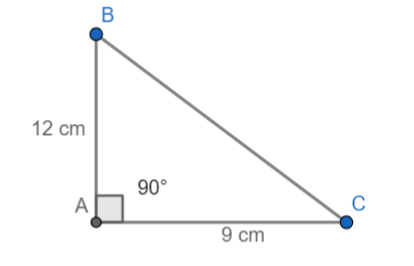Courses
Courses for Kids
Free study material
Offline Centres
MoreLast updated date: 30th Nov 2023
Total views: 379.8k
Views today: 5.79k

# $\vartriangle ABC$ is right-angled at $\angle A$. Find tan, sin and cos of angles B and C in the following case.AB = 12 cm, AC = 9 cm.Verified
379.8k+ views
Hint: The question contains a right-angled triangle, so we can use the trigonometric ratios of sides of the triangle to solve the question. The sine of an angle is defined by $\dfrac{Opposite\text{ }side\text{ }of\text{ }an\text{ }angle}{hypotenuse}$. Similarly, we can use the trigonometric ratios to find the cosine and tangent of the angles.

Given that we have to find the sin, cos and tan of a given right-angled triangle, which is right-angled at $\angle A$. The length of sides AB and AC are also given as 12 cm and 9 cm respectively. We can analyze the question with the help of the following diagram.We are asked to find sinB, cosB, tanB, sinC, cosC and tanC.
We know that the formula of sin is $\dfrac{Opposite\text{ }side\text{ }of\text{ }an\text{ }angle}{hypotenuse}$.
Here the hypotenuse is not given, so we can find the hypotenuse using Pythagoras theorem.
Let us assume the hypotenuse to be x. Therefore, we get,
${{x}^{2}}={{12}^{2}}+{{9}^{^{2}}}$
$\Rightarrow 144+81=225$
${{x}^{2}}=225$
$x=\pm 15$
Length of hypotenuse cannot be negative. So, we can take the length of the hypotenuse is 15 cm.
Now, we can find sinB and sinC using the below formula,
$\sin B=\dfrac{Opposite\text{ }side\text{ }of\text{ }angle\text{ }B}{hypotenuse}$
The opposite side of angle B is 9 cm and the hypotenuse is 15 cm. Substituting these values, we get,
$\sin B=\dfrac{9}{15}=\dfrac{3}{5}$
$\sin C=\dfrac{Opposite\text{ }side\text{ }of\text{ }angle\text{ C}}{hypotenuse}$
The opposite side of angle C is 12 cm and the hypotenuse is 15 cm. Substituting these values, we get,
$\sin C=\dfrac{12}{15}=\dfrac{4}{5}$
Now we can find the angles cosB and cosC using the below formula,
$\cos B=\dfrac{Adjacent\text{ }side\text{ }of\text{ }angle\text{ }B}{hypotenuse}$
The adjacent side of angle B is 12 cm and the hypotenuse is 15 cm. Substituting these values, we get,
$\cos B=\dfrac{12}{15}=\dfrac{4}{5}$
$\cos C=\dfrac{Adjacent\text{ }side\text{ }of\text{ }angle\text{ C}}{hypotenuse}$
The adjacent side of angle C is 9 cm and the hypotenuse is 15 cm. Substituting these values, we get,
$\cos C=\dfrac{9}{15}=\dfrac{3}{5}$
Now we can find the angles tanB and tanC using the below formula,
$\tan B=\dfrac{Opposite\text{ }side\text{ }of\text{ }angle\text{ }B}{Adjacent\text{ }side\text{ }of\text{ }angle\text{ }B}$
The opposite side of angle B is 9 cm and the adjacent side is 12 cm. Substituting these values, we get, $\tan B=\dfrac{9}{12}=\dfrac{3}{4}$
$\tan C=\dfrac{Opposite\text{ }side\text{ }of\text{ }angle\text{ C}}{Adjacent\text{ }side\text{ }of\text{ }angle\text{ C}}$
The opposite side of angle C is 12 cm and the adjacent side is 9 cm. Substituting these values, we get,
$\tan C=\dfrac{12}{9}=\dfrac{4}{3}$
Therefore, we have found out the angles as$\sin B=\dfrac{3}{5}$ , $\sin C=\dfrac{4}{5}$, $\cos B=\dfrac{4}{5}$, $\cos C=\dfrac{3}{5}$, $\tan B=\dfrac{3}{4}$, $\tan C=\dfrac{4}{3}$.

Note: The length of the side of a triangle cannot be negative. So, we ignored the negative length and considered the positive one only. This question is very simple, but the most common mistake that can be committed here is by not drawing the diagram and calculating the angles mentally. This will lead to the wrong answer as well as create confusion in the students' mind. So to avoid this, always draw the diagram and then proceed.